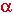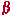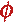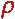Math & Science Home | Proficiency Tests | Mathematical Thinking in Physics | Aeronauts 2000

 CONTENTS Introduction Fermi's Piano Tuner Problem How Old is Old? If the Terrestrial Poles were to Melt... Sunlight Exerts Pressure Falling Eastward What if an Asteroid Hit the Earth Using a Jeep to Estimate the Energy in Gasoline How do Police Radars really work? How "Fast" is the Speed of Light? How Long is a Light Year? How Big is a Trillion? "Seeing" the Earth, Moon, and Sun to Scale Of Stars and Drops of Water If I Were to Build a Model of the Cosmos... A Number Trick Designing a High Altitude Balloon Pressure in the Vicinity of a Lunar Astronaut Space Suit due to Outgassing of Coolant Water Calendar Calculations Telling Time by the Stars - Sidereal Time Fields, an Heuristic Approach The Irrationality ofThe Irrationality ofThe Number (i)i Estimating the Temperature of a Flat Plate in Low Earth Orbit Proving that (p)1/n is Irrational when p is a Prime and n>1 The Transcendentality ofIdeal Gases under Constant Volume, Constant Pressure, Constant Temperature and Adiabatic Conditions Maxwell's Equations: The Vector and Scalar Potentials A Possible Scalar Term Describing Energy Density in the Gravitational Field A Proposed Relativistic, Thermodynamic Four-Vector Motivational Argument for the Expression-eix=cosx+isinx Another Motivational Argument for the Expression-eix=cosx+isinx Calculating the Energy from Sunlight over a 12 hour period Calculating the Energy from Sunlight over actual full day Perfect Numbers-A Case Study Gravitation Inside a Uniform Hollow Sphere Further note on Gravitation Inside a Uniform Hollow Sphere Pythagorean Triples Black Holes and Point Set Topology Additional Notes on Black Holes and Point Set Topology Field Equations and Equations of Motion (General Relativity) The observer in modern physics A Note on the Centrifugal and Coriolis Accelerations as Pseudo Accelerations - PDF File On Expansion of the Universe - PDF File

##### Gravitation Inside A Uniform Hollow Sphere

The gravitational force inside a hollow sphere shell of uniform areal mass density is everywhere equal to zero, and may be proved by the following argument:

Let the sphere have a radius a. Place a point P inside the sphere at a distance r from the center where r < a; i.e., r is strictly less than a. Draw a line through P to intersect the sphere at two opposite points. Call these pointsand. Let the distance from P tobe r1, and the distance from P tobe r2.

Now place a differential area dAat, and project straight lines through P to acquire its image dAat. These two areas subtend a solid angle dat P. Let the sphere have areal mass density(kg/m2). Then the net differential attraction dF of dAand dAat P directed towardis just

dF =( dA/r12 - dA/r22).

But dA= r12 d, and dA= r22 dby definition of the solid angle. Thus,

dF =((r12 d)/r12 - (r22 d)/r22) = 0.

This result is true for all choices of dAand dA. The gravitational force within the sphere is everywhere equal to zero.